Latest Banking jobs   »

# Quantitative Aptitude Quiz For RBI Grade B Phase 1 2023 -15th March

(a) x > y
(b) x ≥ y
(c) x < y
(d) x ≤ y
(e) x = y or no relation can be established between x and y.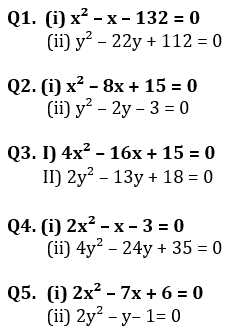Directions (6 – 10): In each of these questions, two equations (I) and (II) are given. You have to solve both the equations and give answer
(a) if x>y
(b) if x≥y
(c) if x<y
(d)if x ≤y
(e) if x = y or no relation can be established between x and y.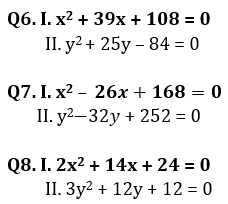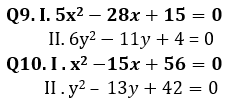Direction (11-15): In the following questions, two equations numbered I and II are given. You have to solve both the equations and give answers among the following options.
(a) if x>y
(b) if x≥y
(c) if x<y
(d) if x ≤y
(e) if x=y or the relationship cannot be established.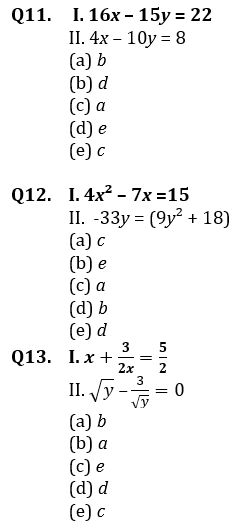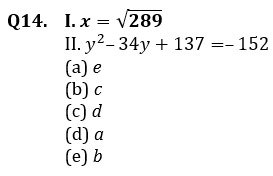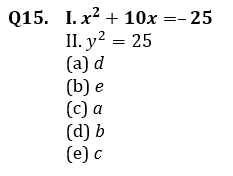Solutions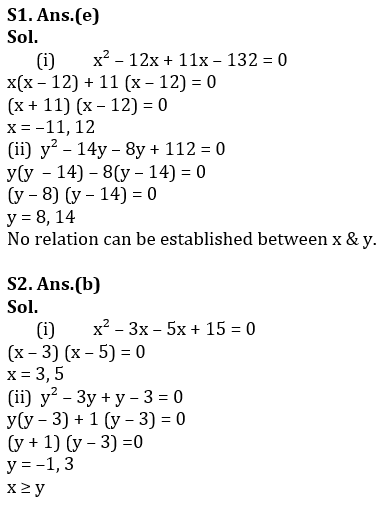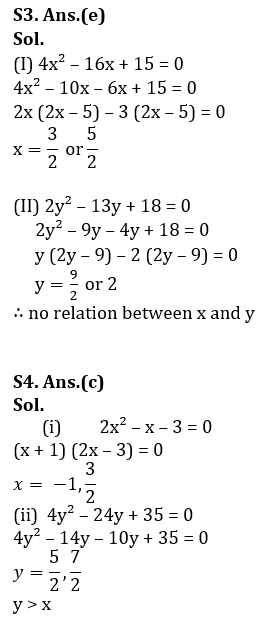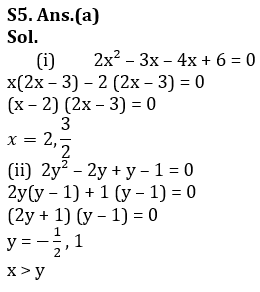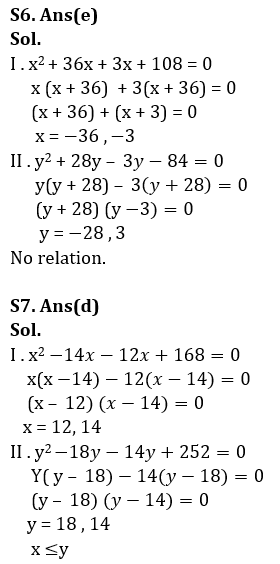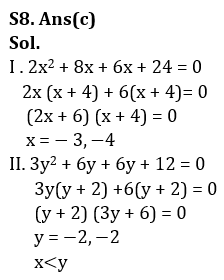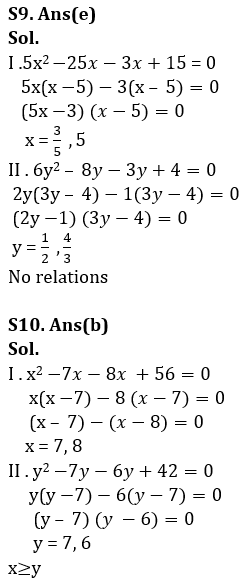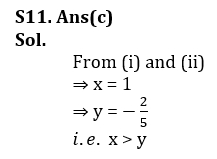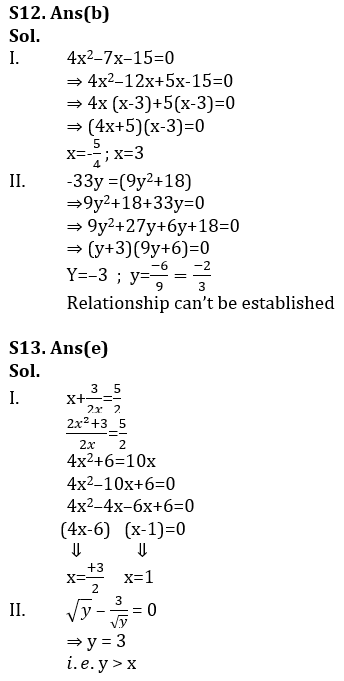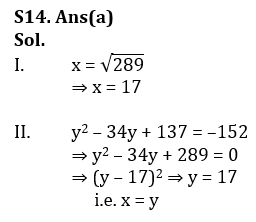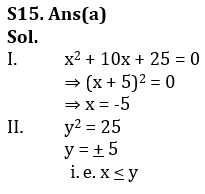## FAQs

### How many sections are there in the RBI Grade B Phase 1 Exam?

There are 4 sections in the RBI Grade B Phase 1 Exam i.e. English Language, General Awareness, Quantitative Aptitude & Reasoning.

#### Congratulations!Union Budget 2023-24: Free PDF